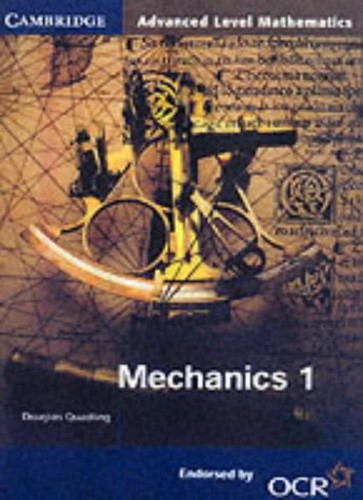# Mechanics 1 for OCR by Douglas Quadling

In Stock
\$8.39
This book is part of the Cambridge Advanced Level Mathematics series, written specifically for the OCR modular mathematics syllabus. Each book or half-book corresponds exactly to one module. Mechanics 1 introduces Newton's laws and has chapters on forces, kinematics and momentum.
Only 1 left

## Mechanics 1 for OCR Summary

### Mechanics 1 for OCR by Douglas Quadling

This book is part of the Cambridge Advanced Level Mathematics series, written specifically for the OCR modular mathematics syllabus. Each book or half-book corresponds exactly to one module. Mechanics 1 introduces Newton's laws and has chapters on forces, kinematics and momentum. Each chapter starts with a short list of learning objectives, and mathematical ideas are explained carefully and clearly, with many worked examples. There are plenty of exercises throughout, along with revision exercises and mock exam papers - all written by experienced examiners.

### Why buy from World of BooksOur excellent value books literally don't cost the earthFree delivery in the USEvery used book bought is one saved from landfill

Introduction; 1. Velocity and acceleration; 2. Force and motion; 3. Vertical motion; 4. Resolving forces; 5. Friction; 6. Objects moving vertically under gravity; 7. Newton's third law; 8. Momentum; 9. Combining and splitting forces; 10. Forces in equilibrium; 11. General motion in a straight line; Revision exercises; Mock examination papers; Answers; Index

GOR001239944
Mechanics 1 for OCR by Douglas Quadling